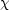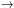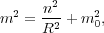VI. EXOTIC CANDIDATES

Although the neutralino and SUSY are well-motivated, other particle candidates for dark matter also exist. The axion is a particle proposed in 1977 by Roberto Peccei and Helen Quinn to solve the so-called "strong-CP problem."  In a nutshell, the strong force Lagrangian contains a term that can give an arbitrarily large electric dipole moment to the neutron; since no electric dipole moment for the neutron has ever been observed, Peccei and Quinn postulated that a new symmetry prevents the appearance of such a term (much like a gauge symmetry keeps the photon massless). They further theorized that this symmetry is slightly broken which leads to a new, very light scalar particle, the axion. Although this particle is extremely light (theories place its mass in the µeV range), it can exist in sufficient numbers to act as cold dark matter. Since axions should couple to photons, axions can be searched for with precisely tuned radio frequency (RF) cavities; inside the magnetic field of an RF cavity the axion can be converted into a photon which shows up as excess power in the cavity. And in a unique blend of particle and astro-physics, limits on axions have been placed through observations of red giant stars; axions, if they existed, would offer another cooling mechanism which can be constrained by studying how quickly red giant stars cool.  Although the axion has never been observed directly, several experiments such as ADMX and CARRACK are continuing the search and setting new limits on axion parameters. [39, 40]

If axions exist and SUSY is also correct, then the axino (the supersymmetric partner of the axion) is by a wide margin the LSP; neutralinos would decay into axinos througha +.  However, axinos would also act as a significant source of hot dark matter and thus could not compose the bulk of the dark matter.

One final exotic particle candidate for dark matter comes from theories of extra spatial dimensions. The idea that our universe could have extra spatial dimensions began in the 1920s with Theodor Kaluza and Oscar Klein; by writing down Eisntein's general theory of relativity in five dimensions, they were able to recover four dimensional gravity as well as Maxwell's equations for a vector field (and an extra scalar particle that they didn't know what to do with). [42, 43] Klein explained the non-observation of this extra fifth dimension by compactifying it on a circle with an extremely small radius (something like 10-35 cm so that it is completely non-observable). Kaluza-Klein theories (as they came to be known) were an initial quest for a grand unified theory; however, the emergence of the weak and strong nuclear forces as fundamental forces of nature relegated this type of approach to unification to the drawing board. However, in the late 1990s two new scenarios with extra dimensions appeared. Arkani-Hamed, Dvali, and Dimpoulos tried to solve the hierarchy problem by assuming the existence of large extra dimensions (initially on the scale of mm or smaller); they made the bold assertion that the electroweak scale is the only fundamental scale in nature and that the Planck scale appears so small due to the presence of these extra dimensions.  Lisa Randall and Raman Sundum, on the other hand, proposed infinitely large extra dimensions that were unobservable at low energies; gravity, they explained, was weak precisely because it was the only force that could "leak-out" into this extra dimension. 

What do extra dimensions have to do with candidates for dark matter? In theories in which extra dimensions are compactified, particles which can propagate in these extra dimensions have their momenta quantized as p2 ~ 1 / R2, where p is the particle's momentum and R is the size of the extra dimension. Therefore, for each particle free to move in these extra dimensions, a set of fourier modes, called Kaluza-Klein states, appears:(6)

where R is the size of the compactified extra dimension, m0 is the regular standard model mass of the particle, and n is the mode number. Each standard model particle is then associated with an infinite tower of excited Kaluza-Klein states. If translational invariance along the fifth dimension is postulated, then a new discrete symmetry called Kaluza-Klein parity exists and the Lightest Kaluza-Klein particle (LKP) can actually be stable and act as dark matter. In most models the LKP is the first excitation of the photon; in the Universal Extra Dimensions (UED) model, if the LKP has mass between 500 and 1200 GeV, then the LKP particle can exist in sufficient numbers to act as the dark matter.  Additional motivations for extra dimensional theories of dark matter include proton stability and the cancellation of gauge anomalies from three generations of fermions. 

Many other theories have been proposed to account for the universe's dark matter, most of which are not as promising as those already discussed. These include Q-balls, mirror particles, WIMPzillas, and branons among many others. [48, 49, 50] However, the neutralino remains the most studied and most theoretically motivated dark matter candidate. In the next section we will discuss how particles like the neutralino (the SUSY LSP) can be produced in the early universe and how to determine if they exist today in sufficient density to act as the dark matter.